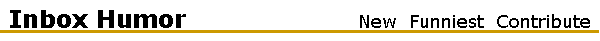### Categories

• Camping
• Children
• Computers
• Dr. Seuss
• Math
• Philosophy
• Relaxation
• School
• Stay in Bed Today
• Work
• ## Wealth & Knowledge... Mathematically Stated

After applying some simple algebra to some trite phrases and cliches a new understanding can be reached of the secret to wealth and success.

Here it goes.

Knowledge is Power
Time is Money and as every engineer knows,BR> Power is Work over Time.

So, substituting algebraic equations for these time worn bits of wisdom, we get:

K = P (1)
T = M (2)
P = W/T (3)

Now, do a few simple substitutions:
Put W/T in for P in equation (1), which yields:

K = W/T (4)

Put M in for T into equation (4), which yields:

K = W/M (5).

Now we've got something. Expanding back into English, we get:

Knowledge equals Work over Money.

What this MEANS is that:

1. The More You Know, the More Work You Do, and/or
2. The More You Know, the Less Money You Make.

Solving for Money, we get:

M = W/K (6)

Money equals Work Over Knowledge.

From equation (6) we see that Money approaches infinity as Knowledge approaches 0, regardless of the Work done.

What THIS MEANS is:

The More you Make, the Less you Know.

Solving for Work, we get

W = M K (7)

Work equals Money times Knowledge

From equation (7) we see that Work approaches 0 as Knowledge approaches 0.

What THIS MEANS is:

The stupid rich do little or no work
.

Working out the socioeconomic implications of this breakthrough is left as an exercise for the reader.